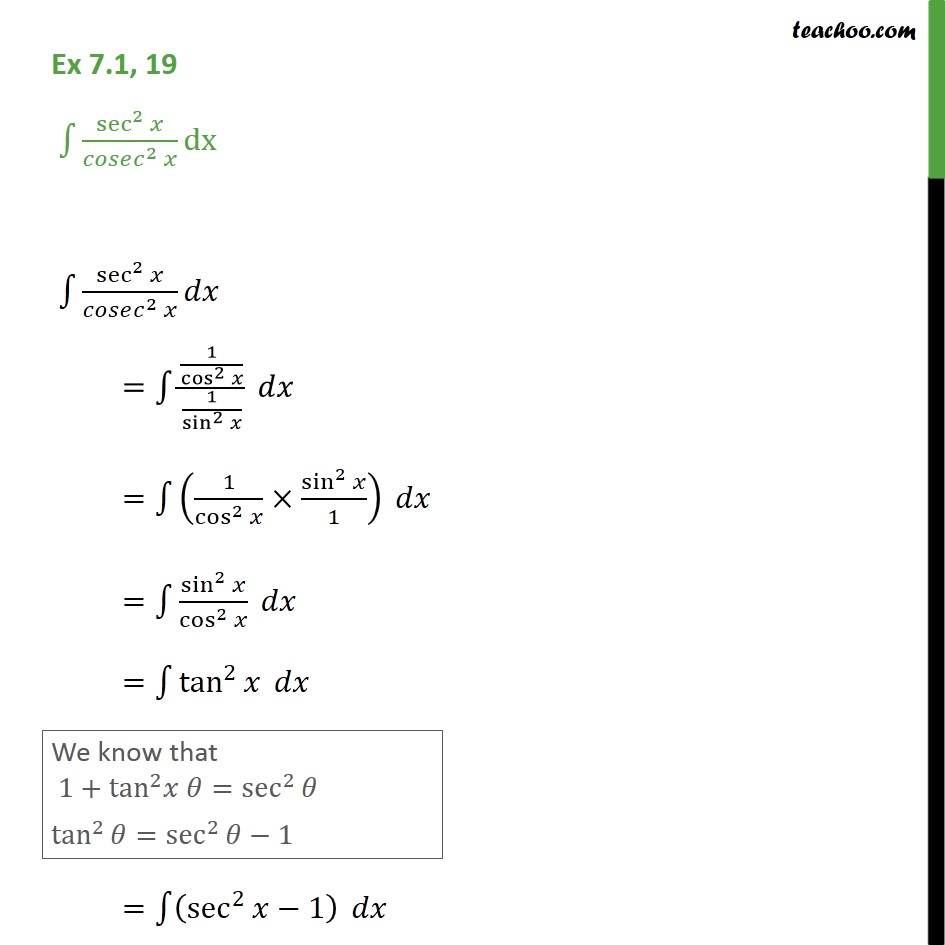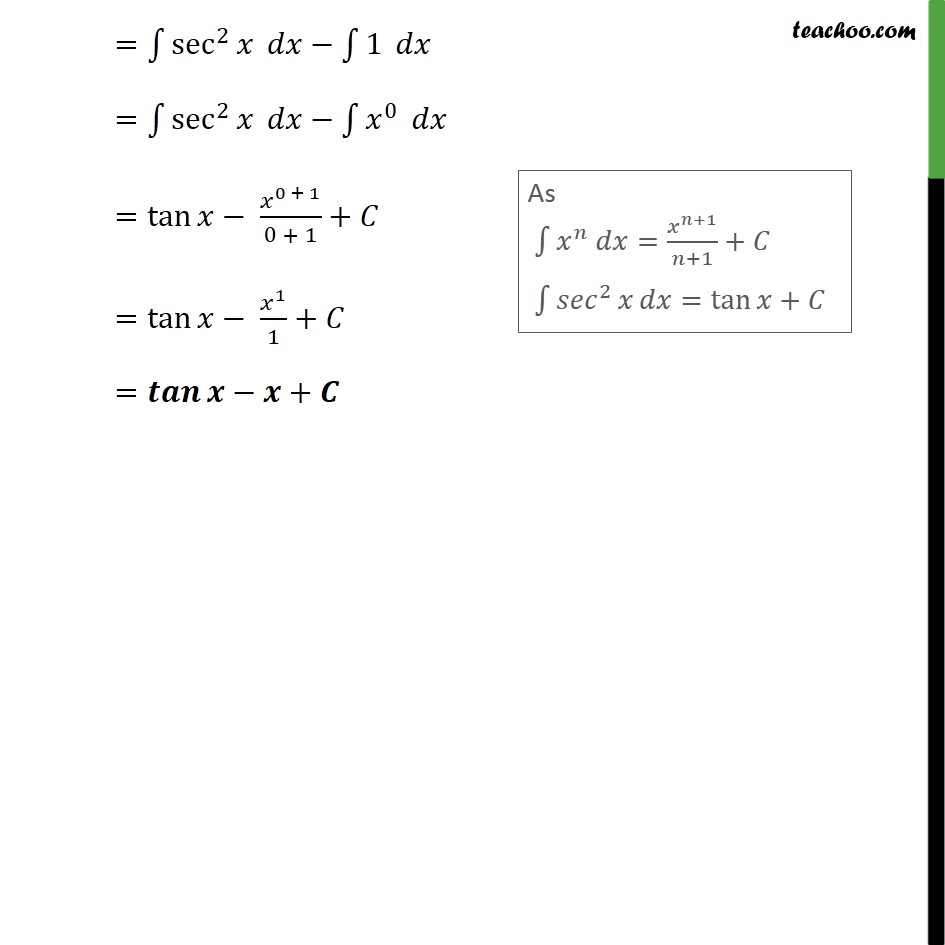Using Trignometric Formulaes

Chapter 7 Class 12 Integrals
Concept wiseIntroducing your new favourite teacher - Teachoo Black, at only ₹83 per month

### Transcript

Ex 7.1, 19 sec 2 2 dx sec 2 2 = 1 cos 2 1 sin 2 = 1 cos 2 sin 2 1 = sin 2 cos 2 = tan 2 = sec 2 1 = sec 2 1 = sec 2 0 = tan 0 + 1 0 + 1 + = tan 1 1 + = +